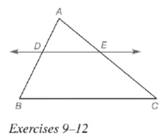Chapter 5.6, Problem 10E### Elementary Geometry for College St...

6th Edition
Daniel C. Alexander + 1 other
ISBN: 9781285195698

#### Solutions

Chapter
Section### Elementary Geometry for College St...

6th Edition
Daniel C. Alexander + 1 other
ISBN: 9781285195698
Textbook Problem
1 views

# Given: D E ↔ ∥ B C , ¯ A D = 6 , D B = 10 , A C = 20 Find: E CTo determine

To find:

EC.

Explanation

Given:

Theorem used:

If a line is parallel to one side of a triangle and intersects other two sides, then it divides these sides proportionally.

Formula used:

If ab=cd then a+bb=c+dd.

Calculation:

Given: DEBC¯

Since if a line is parallel to one side of a triangle and intersects other two sides, then it divides these sides proportionally.

We know that, if ab=cd then a+bb=c+dd.

From the figure, we see that AE+EC=AC

### Still sussing out bartleby?

Check out a sample textbook solution.

See a sample solution

#### The Solution to Your Study Problems

Bartleby provides explanations to thousands of textbook problems written by our experts, many with advanced degrees!

Get Started

#### In Exercises 2932, find the value(s) of x that satisfy the expression. 29. 2x2 + 3x 2 0

Applied Calculus for the Managerial, Life, and Social Sciences: A Brief Approach

#### Calculate y'. 47. y = cosh1(sinh x)

Single Variable Calculus: Early Transcendentals, Volume I

#### Explain how inter-rater reliability is established.

Research Methods for the Behavioral Sciences (MindTap Course List)